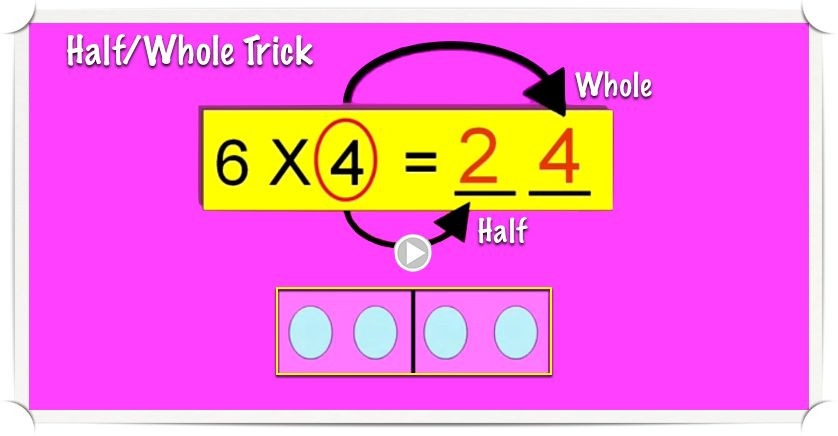##### Print or Share Flyer## 03 NovMath fact mastery: Reaching the fluency finish line

Why use strategies (like Rule, Trick, Chant, and Connection™) to teach Math facts rather than just having students memorize in a traditional rote manner? The Factivation!® strategies utilize existing schema. They apply meaning to each fact, thereby increasing a students' ability to recall the product.

## 25 AugMultiplication trick makes the Sixes facts simple!### Simplify the Sixes facts with the Half-Whole Trick!

This Multiplication trick makes learning the Sixes facts simple! The "Half-Whole" multiplication trick works like this:

### When multiplying six by an even number, the product is HALF of that number (tens place), then the WHOLE number (ones place).

Here's an example: